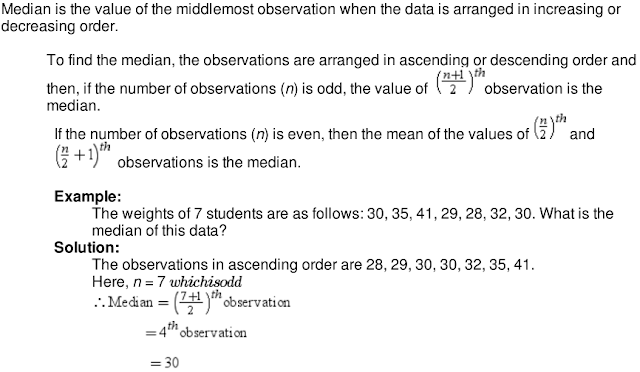## Revision Notes of Chapter 14 Statistics Class 9th Math

Topics in the Chapter
• Different Bar Graphs for Data Representation
• Pictograph and Bar Graph
• Grouped Frequency Distribution Table
• Construction of histogram of different class intervals
• Interpretation of histograms
• Construction of frequency polygons
• Mean of data sets
• Mean of a frequency distribution table
• Median
Bar Graphs for Data Representation

Data can be represented graphically in the following ways:
(i) Bar Graph(ii) Histogram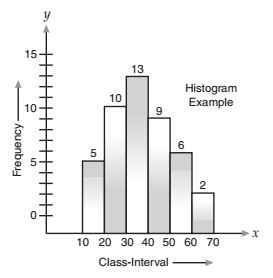(iii) Frequency polygon.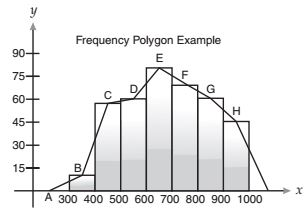Pictograph and Bar Graph

• In a pictograph, pictures of objects are used for representing data. Tally marks cannot be used for representing huge numbers. However, these numbers can be represented with the help of pictographs.
• Data can also be represented by using bar diagram or bar graph. In a bar graph, bars of uniform width are drawn horizontally or vertically. These bars are placed at equal distance from each other. The length of each bar gives the required information.
• The data in an unorganised form is called raw data. In order to draw meaningful inferences from a data, we need to organise the data systematically.

Grouped Frequency Distribution Table

There are two ways to group the data to make frequency distribution table. These are as follows:
(i) Inclusive method: Discontinuous form
(ii) Exclusive method : Continuous form

The classes can be defined in inclusive method as 1 - 10, 11 - 20, 21 - 30 and 31 - 40. Here, both limits are inclusive in each class.

In exclusive method, we take the class intervals as 0 – 10, 10 – 20, 20 – 30. The observations which are more than 0 but less than 10 will come under the group 0 – 10. The numbers which are more than 10 but less than 20 will come under the group 10 – 20 and so on. Here, the common observation will belong to the higher class, i.e. 10 will be included in the class interval 10 – 20 and similarly we follow this for the other observations also.

For example, the ages of some residents of a particular locality are given as follows:
7, 28, 30, 32, 18, 19, 37, 36, 14, 27, 12, 8, 17, 24, 22, 2, 21, 5, 21, 36, 38, 25, 10, 25, 9.
Frequency distribution table can be drawn as follows:Few points to be remembered while choosing class intervals
(i) Classes should not be overlapping and all values or observations should be covered in these classes.
(ii) The class size for all classes should be equal.
(iii) The number of class intervals is normally between 5 and 10.
(iv) Class marks and class limits should be taken as integers or simple fractions.

Construction of histogram of different class intervals

A histogram is a graphical representation of grouped data.The histogram of the given data can be drawn as follows:Interpretation of histograms

The interpretation of data represented by a histogram can be done by looking at it.

Example:

The following histogram shows the production of food grains over a period of time.What is the total production of food grains from 2004 to 2009?
In which periods were the production of food grains the highest and the lowest?

Solution:

The total production of food grains from 2004 to 2009 can be ascertained by adding the heights of the class intervals 2004–2007 and 2007–2009.
∴ Total production of food grains from 2004 to 2009 = 7000 tonnes + 6000 tonnes = 13000 tonnes
It is clear from the histogram that the bar corresponding to the class interval 2004–2007 is the tallest, and that corresponding to the class interval 2009–2010 is the shortest.
So, the production of food grains was the highest in the period 2004–2007 and the lowest in the period 2009–2010

Construction of frequency polygons

A frequency polygon is a continuous curve obtained by plotting and joining the ordered pairs of class marks and their corresponding frequencies.

There are two ways to construct a frequency polygon.

(i) The frequency polygon for a grouped data is drawn by first drawing its histogram and then by joining the mid-points of the top of bars and the mid-points of the classes preceding and succeeding the lowest and highest class respectively.

(ii) One other way of drawing a frequency polygon is by plotting and joining the ordered pairs of class mark and their corresponding frequencies with the mid-points of the classes preceding and succeeding lowest and highest class respectively.

Mean of data sets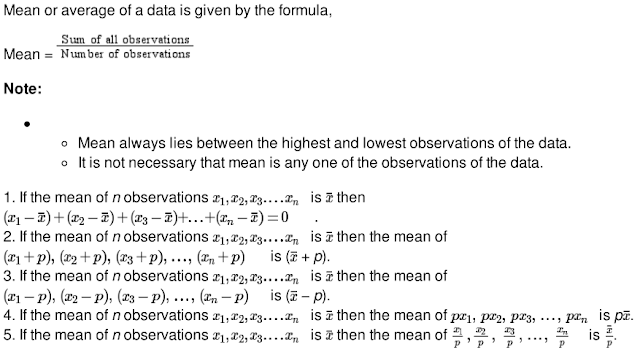Mean of a frequency distribution table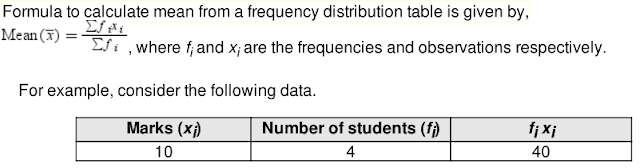Median